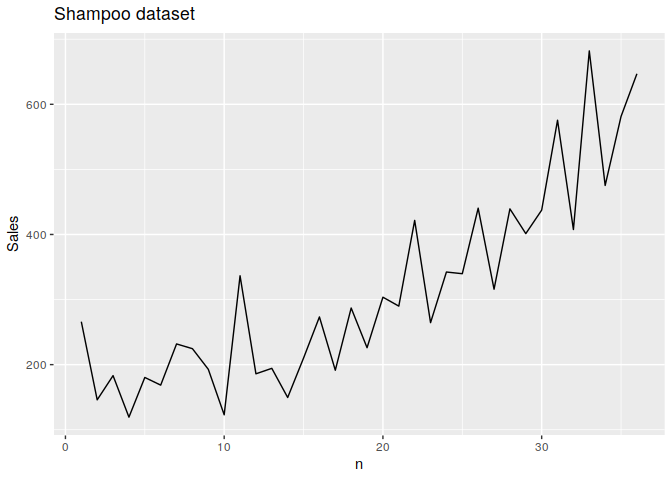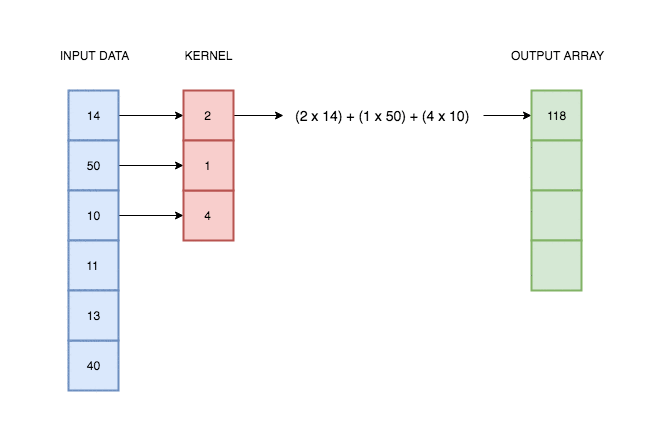# Time Series & torch #1 - Training a network to compute moving averageIn the previous year, I published a post, which as I hoped, was the first tutorial of the series describing how to effectively use PyTorch in Time Series Forecasting. Recently, a new exciting R package was submitted on CRAN. This great news was officially announced on the RStudio AI Blog. Yes, you mean right - the R port of PyTorch - called simply `torch` came into play. This encouraged me to reactivate my series, but in this time with both R and Pythonic versions. I’ll begin with rewriting my previous-year post.

### 1. Getting the data

In PyTorch version I used a Shampoo sales dataset published by Rob Hyndman in his R package fma (a software appendix for the book Forecasting: Methods and Applications). Instead of installing Hyndman’s lib, we’ll download the dataset from the Web. It’s because this version is already well-foramtted and we’ll avoid additional transformation. First of all, let’s present the `shampoo` dataset.

``````library(ggplot2)
library(dplyr)
library(data.table)
library(torch)
``````
``````shampoo <- read.csv("https://raw.githubusercontent.com/jbrownlee/Datasets/master/shampoo.csv")
setDT(shampoo)
shampoo[, n := 1:.N]
``````

### 2. Simple visualization

``````print(head(shampoo))
``````
``````##    Month Sales n
## 1:  1-01 266.0 1
## 2:  1-02 145.9 2
## 3:  1-03 183.1 3
## 4:  1-04 119.3 4
## 5:  1-05 180.3 5
## 6:  1-06 168.5 6
``````
``````ggplot(shampoo) +
geom_line(aes(x = n, y = Sales)) +
ggtitle("Shampoo dataset")
``````In this plot we can see an increasing trend, but in this excercise, data characterics make no diffeence for us.

### 3. 1-d convolution in PyTorch: lightning-quick intro (or reminder)

In the case of univariate time series, one-dimensional convolution is a sliding window applied over time series, an operation which consist of multiplications and additions. It was intuitively illustrated on the gif below.**Source: https://blog.floydhub.com/reading-minds-with-deep-learning/**

As you can see, output depend on input and kernel values. Defining proper kernel, we can apply the operation we want. For example, using a (0.5, 0.5) kernel, it will give us a two-element moving average. To test that, let’s do a simple experiment.

### 4. Computing moving average with `data.table`

Among its many features, `data.table` offers a set of ‘fast’ functions (with names prefixed with f). One example of this great stuff is a `frollmean` functions, which computes moving average. We use a standard `head` function as well, to limit the output. What is worth to mention is that a NA appeared in the first row. It’s because we can’t compute moving avearge for the first element if we haven’t added any padding on the beginning of the array; moreover, `frollmean` keeps the input’s length, so the first element has no value.

``````ts <- shampoo\$Sales

ts %>%
frollmean(2) %>%
``````
``````##       NA 205.95 164.50 151.20 149.80 174.40 200.15 228.15 208.65 157.85
``````

### 5. Computing moving average with `torch`

Now, let’s reproduce this result using 1-dimensional convolution from `torch`.

``````ts_tensor <- torch_tensor(ts)\$reshape(c(1, 1, -1))
``````

Let’s stop here for a moment. If you are not familiar with deep learning frameworks, you would be quite confused because of this `reshape` operation. What did we do above? We created a 3-dimensional tensor; each number in `reshape` function describes respectively:

1. number of samples
2. number of channels
3. length of time series

Meaning of this values requires some explanation.

1. Number of samples is the number of time series we are working on. As we want to perform computations for one time series only, the value must equal one.
2. Number of channels is is the number of features or (independent) variables. We don’t have any parallel variables containing information about, say, temperature or population. It’s clear that this value must equal one too.
3. Length of time series. Accordingly to `torch` tensor reshaping convention, minus one means infer value for this dimension. If one-dimensional time series length has 36 elements, after reshaping it to three-dimensional tensor with number_of_samples = 1 and number_of_channels = 1, the last value will be equal to 36.

We have to do the same with the kernel.

``````kernel <- c(0.5, 0.5)
kernel_tensor <- torch_tensor(kernel)\$reshape(c(1, 1, -1))
torch_conv1d(ts_tensor, kernel_tensor)
``````
``````## torch_tensor
## (1,.,.) =
##  Columns 1 to 7  205.9500  164.5000  151.2000  149.8000  174.4000  200.1500  228.1500
##
## Columns 8 to 14  208.6500  157.8500  229.7000  261.2000  190.1000  171.9000  179.8000
##
## Columns 15 to 21  241.7000  232.3500  239.2000  256.5000  264.8000  296.7500  355.7500
##
## Columns 22 to 28  343.0500  303.4000  341.0000  390.0500  378.1500  377.6000  420.3000
##
## Columns 29 to 35  419.3500  506.4500  491.5500  544.8000  578.6500  528.3000  614.1000
## [ CPUFloatType{1,1,35} ]
``````

As we can observe, the result is identical with values returned by `frollmean` function. The only difference is lack of NA on the beginning.

### 6. Learning a network, which computes moving average

Now, let’s get to the point and train the network on the fully controllable example. I’ve called in this manner to distinguish it from the real-life ones. In most cases, when we train a machine learning model, we don’t know the optimal parameter values. We are just trying to choose the best ones, but have no guarantee that they are globally optimal. Here, the optimal kernel value is known and should equal [0.2, 0.2, 0.2, 0.2, 0.2].

``````X_tensor <-  torch_tensor(ts)\$reshape(c(1,1,-1))
``````

In the step below, we are preparing targets (labels), which equals to the five-element moving average.

``````y <- frollmean(ts, 5)
y <- y[-(1:4)]
y_tensor <-  torch_tensor(y)\$reshape(c(1,1,-1))
y_tensor
``````
``````## torch_tensor
## (1,.,.) =
##  Columns 1 to 7  178.9200  159.4200  176.6000  184.8800  199.5800  188.1000  221.7000
##
## Columns 8 to 14  212.5200  206.4800  197.8200  215.2600  202.6200  203.7200  222.2600
##
## Columns 15 to 21  237.5600  256.2600  259.5800  305.6200  301.1200  324.3800  331.6000
##
## Columns 22 to 28  361.7000  340.5600  375.5200  387.3200  406.8600  433.8800  452.2200
##
## Columns 29 to 32  500.7600  515.5600  544.3400  558.6200
## [ CPUFloatType{1,1,32} ]
``````

We are building a one-layer convolutional neural network. It’s good to highlight, that we don’t use any nonlinear activation function. Last numerical value describes the length of the kernel, padding = 0 means that we don’t add any padding to the input, so we have to expect that output will be “trimmed”.

``````net <- nn_conv1d(1, 1, 5, padding = 0, bias = FALSE)
``````

Kernel is already initialized with, assume it for simplicity, random values.

``````net\$parameters\$weight
``````
``````## torch_tensor
## (1,.,.) =
##  -0.0298  0.1094 -0.4210 -0.1510 -0.1525
## [ CPUFloatType{1,1,5} ]
``````

We can perform a convolution operation using this random value, calling net\$forward() or simply net(). This two operations are equivalent.

``````net(X_tensor)
``````
``````## torch_tensor
## (1,.,.) =
##  Columns 1 to 7 -114.5778  -87.4777 -129.1170 -124.0212 -147.8481 -122.0550 -133.4026
##
## Columns 8 to 14 -116.5216 -191.6899  -97.2734 -126.1265 -120.6398 -148.3641 -169.2148
##
## Columns 15 to 21 -134.7664 -188.4784 -159.5273 -219.7331 -199.5979 -246.9963 -177.3924
##
## Columns 22 to 28 -246.2201 -228.1574 -273.1713 -222.5049 -290.8464 -284.1429 -302.4402
##
## Columns 29 to 32 -371.9796 -297.1908 -420.1493 -324.1110
## [ CPUFloatType{1,1,32} ]
``````

We are initializing an optimizer object. I highly encourage you to experiment and start with SGD which may do not converge.

``````# optimizer <- optim_sgd(net\$parameters, lr = 0.01)
optimizer <- optim_adam(net\$parameters, lr = 0.01)
``````

Here, he have only one example so it does not make sense to divide training into epochs.

``````running_loss <-  0.0

for (iteration in 1:2000) {

# Forward propagation
outputs <-  net(X_tensor)

# Mean squared error
loss_value <- torch_mean((outputs - y_tensor)**2)

loss_value\$backward()

# Changing network parameters with optimizer
optimizer\$step()

# Extracting loss value from tensor
running_loss <-  running_loss + loss_value\$item()

flat_weights <- net\$parameters\$weight %>%
as_array() %>%
as.vector()

if (iteration %% 50 == 0) {
print(glue::glue("[{iteration}] loss: {loss_value\$item()}"))
print(flat_weights)
}
}
``````
``````##  loss: 795.017639160156
##   0.3119572  0.4480094 -0.0774434  0.1887493  0.1892590
##  loss: 627.464172363281
##   0.30481237  0.42822435 -0.07718747  0.17363353  0.18184586
##  loss: 546.570983886719
##   0.3097025  0.4179998 -0.0630119  0.1692921  0.1865403
##  loss: 471.807800292969
##   0.31258762  0.40443128 -0.04937108  0.16256894  0.18939941
##  loss: 401.237457275391
##   0.31531987  0.39036036 -0.03479132  0.15607581  0.19235790
##  loss: 337.717254638672
##   0.31756479  0.37616777 -0.01987797  0.15002672  0.19514479
##  loss: 282.553039550781
##   0.319161922  0.362225264 -0.005009139  0.144656733  0.197645336
##  loss: 235.910583496094
##  0.320012957 0.348812759 0.009538475 0.140130043 0.199790746
##  loss: 197.225311279297
##  0.32006672 0.33612481 0.02356522 0.13654210 0.20154381
##  loss: 165.532333374023
##  0.31931198 0.32428458 0.03693568 0.13392988 0.20289351
##  loss: 139.712768554688
##  0.31777066 0.31335631 0.04956749 0.13228267 0.20385022
##  loss: 118.661178588867
##  0.31549129 0.30335727 0.06142059 0.13155238 0.20444071
##  loss: 101.386795043945
##  0.31254151 0.29426861 0.07248778 0.13166353 0.20470326
##  loss: 87.0595397949219
##  0.30900255 0.28604546 0.08278601 0.13252223 0.20468384
##  loss: 75.020133972168
##  0.30496314 0.27862594 0.09234858 0.13402404 0.20443186
##  loss: 64.7659072875977
##  0.3005151 0.2719381 0.1012190 0.1360608 0.2039973
##  loss: 55.9260444641113
##  0.2957492 0.2659062 0.1094460 0.1385261 0.2034285
##  loss: 48.2335586547852
##  0.2907525 0.2604553 0.1170791 0.1413187 0.2027697
##  loss: 41.4970893859863
##  0.2856061 0.2555139 0.1241664 0.1443462 0.2020606
##  loss: 35.5792236328125
##  0.2803833 0.2510171 0.1307523 0.1475262 0.2013350
##  loss: 30.3781261444092
##  0.2751493 0.2469072 0.1368768 0.1507875 0.2006208
##  loss: 25.8145942687988
##  0.2699609 0.2431345 0.1425748 0.1540700 0.1999404
##  loss: 21.8240375518799
##  0.2648661 0.2396567 0.1478763 0.1573242 0.1993102
##  loss: 18.3501605987549
##  0.2599051 0.2364388 0.1528070 0.1605106 0.1987420
##  loss: 15.3419895172119
##  0.2551105 0.2334520 0.1573887 0.1635987 0.1982433
##  loss: 12.7523593902588
##  0.2505079 0.2306734 0.1616401 0.1665655 0.1978179
##  loss: 10.5367918014526
##  0.2461172 0.2280841 0.1655775 0.1693947 0.1974661
##  loss: 8.65341949462891
##  0.2419526 0.2256693 0.1692155 0.1720755 0.1971868
##  loss: 7.06301403045654
##  0.2380237 0.2234169 0.1725675 0.1746014 0.1969763
##  loss: 5.72896862030029
##  0.2343363 0.2213169 0.1756462 0.1769695 0.1968299
##  loss: 4.61755132675171
##  0.2308923 0.2193609 0.1784641 0.1791797 0.1967420
##  loss: 3.69792985916138
##  0.2276909 0.2175417 0.1810337 0.1812342 0.1967065
##  loss: 2.94231581687927
##  0.2247288 0.2158528 0.1833675 0.1831365 0.1967170
##  loss: 2.32577872276306
##  0.2220005 0.2142882 0.1854781 0.1848916 0.1967671
##  loss: 1.82624590396881
##  0.2194988 0.2128422 0.1873784 0.1865052 0.1968507
##  loss: 1.42442286014557
##  0.2172151 0.2115093 0.1890816 0.1879836 0.1969618
##  loss: 1.10348606109619
##  0.2151396 0.2102839 0.1906009 0.1893335 0.1970950
##  loss: 0.849016129970551
##  0.2132619 0.2091608 0.1919495 0.1905621 0.1972449
##  loss: 0.648723244667053
##  0.2115705 0.2081344 0.1931406 0.1916765 0.1974071
##  loss: 0.492226451635361
##  0.2100540 0.2071995 0.1941869 0.1926837 0.1975773
``````

As we can see in this example, algorithm converges and parameter values are becoming close to the true solution, i.e. [0.2, 0.2, 0.2, 0.2, 0.2].

On my blog, you can also find a Python version of this post.